/

### Function Description

Returns an array corresponding to a weighted cubic curve fit to observed quantile values, where the weights correspond to the distance between consecutive points (so that the fit gives greater weight to the tails of the distribution). Requires as inputs an ordered array of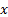values (e.g. expected quantiles, as per MnStandardisedNormalQuantiles and an ordered array of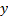values corresponding to the actually observed values.

The curve fit is derived using the Nematrian least squares generalised curve fit MnLeastSquaresGeneralisedCurveFit, (specifically the polynomial version).

The weights,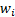, used in this function, if the ordered-values are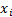and there aresuch values, are: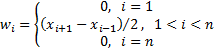This potentially under allows for the two end points. An alternative that gives greater weight to the two end points is MnStandardWeightedCubicQuantileFitInclEnds.

Please bear in mind that a cubic is not always a valid form for a quantile-quantile function to take (e.g. if the slope of the cubic becomes negative anywhere). If you wish to avoid this problem then we suggest fitting a distributional form using e.g. (weighted) maximum likelihood, see e.g. MnProbDistWeightedMLE.

Contents | Prev | Next